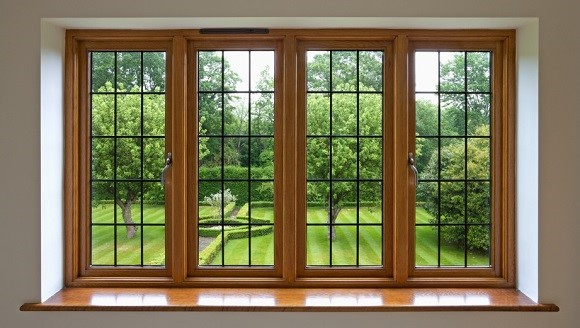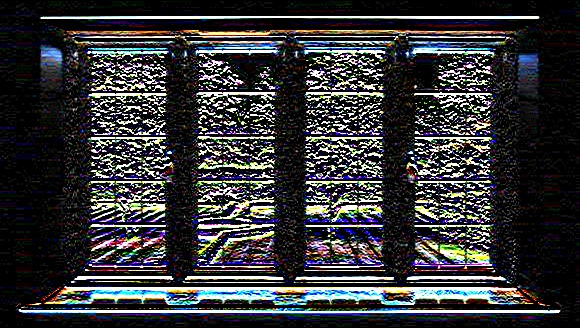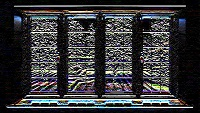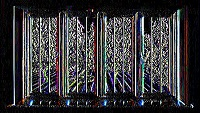# OpenCV - Scharr Operator

Scharr is also used to detect the second derivatives of an image in horizontal and vertical directions. You can perform scharr operation on an image using the method scharr(). Following is the syntax of this method −

```Scharr(src, dst, ddepth, dx, dy)
```

This method accepts the following parameters −

• src − An object of the class Mat representing the source (input) image.

• dst − An object of the class Mat representing the destination (output) image.

• ddepth − An integer variable representing the depth of the image (-1)

• dx − An integer variable representing the x-derivative. (0 or 1)

• dy − An integer variable representing the y-derivative. (0 or 1)

### Example

The following program demonstrates how to apply scharr to a given image.

```import org.opencv.core.Core;
import org.opencv.core.Mat;

import org.opencv.imgcodecs.Imgcodecs;
import org.opencv.imgproc.Imgproc;

public class ScharrTest {

public static void main( String[] args ) {

// Reading the Image from the file and storing it in to a Matrix object
String file ="E:/OpenCV/chap16/sobel_input.jpg";

// Creating an empty matrix to store the result
Mat dst = new Mat();

// Applying Box Filter effect on the Image
Imgproc.Scharr(src, dst, Imgproc.CV_SCHARR, 0, 1);

// Writing the image
Imgcodecs.imwrite("E:/OpenCV/chap16/scharr_output.jpg", dst);

System.out.println("Image processed");
}
}
```

Assume that following is the input image scharr_input.jpg specified in the above program.### Output

On executing it, you will get the following output −

```Image Processed
```

If you open the specified path you can observe the output image as follows −## More scharr Derivatives

On passing different values to the last to parameters (dx and dy) (among 0 and 1) you will get different outputs −

```// Applying scharr on the Image
Imgproc.Scharr(src, dst, -1, 1, 1);
```

Following is a table listing various values for the variables dx and dy of the method scharr() and their respective outputs.

X-derivative Y-derivative Output
0 11 0